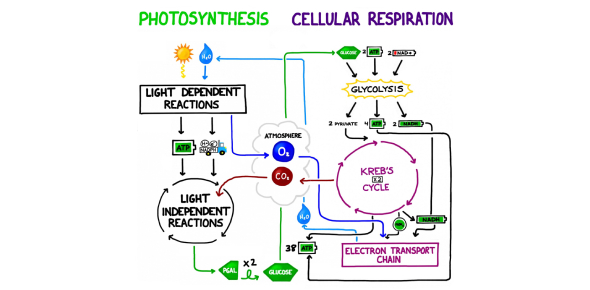# Trivia: Quiz On Photosynthesis And Cellular Respiration!

10 Questions | Total Attempts: 224SettingsCreate your own Quiz.

• 1.
What is one of the reactants for cellular respiration?
• A.

Glucose

• B.

Water

• C.

Carbon dioxide

• D.

Energy

• 2.
What are the products for photosynthesis?
• A.

Sugar + glucose

• B.

Carbon dioxide

• C.

Sugar + oxygen

• D.

Water + energy

• 3.
During respiration, the reactants of photosynthesis are changed into
• A.

H2O, CO2, and energy

• B.

C6H12O6 and O2

• C.

Energy and O2

• D.

C6H12O2 and H2O

• 4.
In cellular respiration what is the correct equation?
• A.

602+C6H12O6+energy -> 6CO2+6H2O

• B.

6CO2+6H2O+energy->6O2+c6H12O6

• C.

6O2+c6H12O6->6CO2+6H2O+energy

• D.

6O2+C6H12O6+energy->6CO2+6H2O

• 5.
How much ATP does aerobic respiration produce?
• A.

2 ATP

• B.

15 ATP

• C.

20 ATP

• D.

36 ATP

• 6.
Which is the correct equation for photosynthesis?
• A.

H2O + O2 -> energy+C6H12O6

• B.

CO2+H2O -> C6H12O6+O2

• C.

C6H12O6+energy -> H2O+O2

• D.

C6H12O6+O2 -> CO2+H2O

• 7.
Cellular respiration occurs in which cell type?
• A.

Plant cells only

• B.

Animal cell only

• C.

Both plants and animal cell

• D.

All but plant cells

• 8.
What molecules belong in the box of the diagram?
• A.

1 transpiration; 2 respiration

• B.

1 chemosynthesis; 2photosynthesis

• C.

1 respiration; 2 photosynthesis

• D.

1 photosythesis; 2 respiration

• 9.
QIn cellular respiration energy can be released by breaking down which molecule?
• A.

Atp

• B.

Carbon doixide

• C.

Glucose

• D.

Water

• 10.
What molecules does aerobic respiration use that anaerobic respiration is missing?
• A.

Oxygen

• B.

Glucose

• C.

Exercise

• D.

Light

Related TopicsBack to top
×

Wait!
Here's an interesting quiz for you.